﻿ Is Planck’s Constant - A Cosmological Variable?International Journal of Astronomy

p-ISSN: 2169-8848    e-ISSN: 2169-8856

2013;  2(1): 11-15

doi:10.5923/j.astronomy.20130201.02

### Is Planck’s Constant - A Cosmological Variable?

U. V. S. Seshavatharam1, S. Lakshminarayana2

1Honorary faculty, I-SERVE, Alakapuri, Hyderabad-35, AP, India

2Dept. of Nuclear Physics, Andhra University,Visakhapatnam-03, AP, India

Correspondence to: U. V. S. Seshavatharam, Honorary faculty, I-SERVE, Alakapuri, Hyderabad-35, AP, India.
 Email: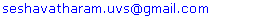Abstract

Within the expanding cosmic Hubble volume, Hubble length can be considered as the gravitational or electromagnetic interaction range. Product of ‘Hubble volume’ and ‘cosmic critical density’ can be called as the ‘Hubble mass’. Based on this cosmic mass unit, authors noticed five peculiar semi empirical relations in atomic, nuclear and cosmic physics. With these applications it is possible to say that – during the cosmic evolution, magnitude of Planck’s constant increases with increasing cosmic time. This may be the root cause of observed cosmic red shifts. By observing the cosmological rate of change in Planck’s constant, the future cosmic acceleration can be verified from the ground based laboratory experiments. With reference to the current concepts of distant and spatial variation of the fine structure ratio, variation of the planck’s constant can be considered for further analysis.

Keywords: Hubble Volume, Cosmic Critical Density, Hubble Mass, Cosmic Thermal Energy Density, Planck’s Constant

Cite this paper: U. V. S. Seshavatharam, S. Lakshminarayana, Is Planck’s Constant - A Cosmological Variable?, International Journal of Astronomy, Vol. 2 No. 1, 2013, pp. 11-15. doi: 10.5923/j.astronomy.20130201.02.

### 1. Introduction

Einstein, more than any other physicist, untroubled by either quantum uncertainty or classical complexity, believed in the possibility of a complete, perhaps final, theory of everything. He also believed that the fundamental laws and principles that would embody such a theory would be simple, powerful and beautiful. Physicists are an ambitious lot, but Einstein was the most ambitious of all. His demands of a fundamental theory were extremely strong. If a theory contained any arbitrary features or undetermined parameters then it was deficient, and the deficiency pointed the way to a deeper and more profound and more predictive theory. There should be no free parameters – no arbitrariness. According to his philosophy, electromagnetism must be unified with general relativity, so that one could not simply imagine that it did not exist. Furthermore, the existence of matter, the mass and the charge of the electron and the proton (the only elementary particles recognized back in the 1920s), were arbitrary features. One of the main goals of a unified theory should be to explain the existence and calculate the properties of matter.
In physics history, for any new idea or observation or new model - at the very beginning – their existence was very doubtful. The best examples were : 1) Existence of atom 2) Existence of quantum of energy 3) Existence of integral nature of angular momentum 4) Existence of wave mechanics 5) Existence of Black holes 6) Black hole radiation and so on. Another best example is/was: Einstein’s cosmological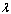term. In this paper authors made an attempt to understand the basic concepts of particle cosmology via five semi empirical applications.
If we write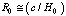as a characteristic cosmic Hubble radius then the characteristic cosmic Hubble volume is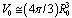The Hubble volume is sometimes defined as a volume of the universe with a commoving size of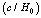. The exact definition varies. Some cosmologists even use the term Hubble volume to refer to the volume of the observable universe. With reference to the cosmic critical density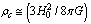and the characteristic cosmic Hubble volume, characteristic cosmic Hubble mass can be expressed as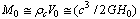(1)
If we do not yet know whether the universe is spatially closed or open, then the idea of Hubble volume or Hubble mass can be used as a tool in cosmology and unification. This idea is very close to the Mach’s idea of distance cosmic back ground. It seems to be a quantitative description to the Mach’s principle. In understanding the basic concepts of unification of the four cosmological interactions, the cosmic radiuscan be considered as the infinite range of the gravitational or electromagnetic interaction.
Within the Hubble volume it is noticed that: 1) Each and every point in free space is influenced by the Hubble mass. 2) Hubble mass plays a vital role in understanding the properties of electromagnetic and nuclear interactions and 3) Hubble mass plays a key role in understanding the geometry of the universe. The current value of the Hubble’s constant is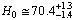Km/sec/Mpc[2,3]. Thus the magnitude of the present cosmic Hubble mass can be given as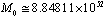Kg.

### 2. Five Peculiar Applications

#### 2.1. Application-1

In physics, the fine-structure ratio (usually denoted by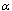) is a fundamental physical constant, namely the coupling constant characterizing the strength of the electromagnetic interaction. Being a dimensionless quantity, it has constant numerical value in all systems of units. The most precise value ofobtained experimentally (as of 2012) is based on a measurement of ‘Linde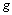factor’ using a ‘one-electron’ so-called ‘quantum cyclotron’ apparatus, together with a calculation via the theory of QED. This measurement ofhas a precision of 0.25 parts per billion.
If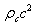is the present cosmic critical energy density and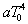is the present cosmic thermal energy density, it is noticed that,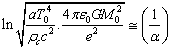(2)
This is a very peculiar relation and constitutes theand. Note that, from unification point of view, till today role of dark energy or dark matter is unclear and undecided. Their laboratory or physical existence is also not yet confirmed. In this critical situation this application can be considered as a key tool in particle cosmology. Note that large dimensionless constants and compound physical constants reflect an intrinsic property of nature. At present if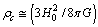, independent of the gravitational constant, relation (2) takes the following form.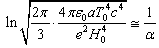(3)
At present if observed CMBR temperature is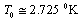, obtained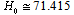Km/sec/Mpc. After simplification, it can be interpreted as follows. Total thermal energy in the present Hubble volume can be expressed as,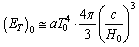(4)
If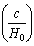is the present electromagnetic interaction range, then the present electromagnetic potential can be expressed as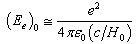(5)
Now inverse of the present fine structure ratio can be expressed as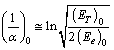(6)
Here, in RHS, denominator ‘2’ may be a representation of the total thermal energy in half of the present Hubble volume. In this way, in a unified manner, the present fine structure ratio can be fitted. From this relation it is possible to say that, cosmological rate of change in fine structure ratio,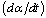may be considered as an index of the future cosmic acceleration. Many physicists think its possible variation and experiments are in progress. Dirac proposed about the variation of the gravitational constant[6,7]. Compared to the concept of ‘variation of gravitational constant’ – ‘variation of fine structure ratio’ seems to be testable in ground based spectroscopic observations easily. While the fine-structure constant is known to approach 1/128 at interaction energies above 80 GeV, physicists have pondered for many years whether the fine-structure constant is in fact a constant, i.e., whether or not its value differs by location and over time. Specifically, a varyinghas been proposed as a way of solving problems in cosmology and astrophysics.
More recently, theoretical interest in varying constants (not just) has been motivated by string theory and other such proposals for going beyond the Standard Model of particle physics. The first experimental tests of this question examined the spectral lines of distant astronomical objects and the products of radioactive decay in the Oklo natural nuclear fission reactor. The findings were consistent with no change. In October 2011 Webb et al. reported a variation independent on both redshift and spatial direction. Till today from ground based laboratory experiments no variation was noticed in the magnitude of the fine structure ratio. Future experiments and observations may reveal the real picture.

#### 2.2. Application-2

If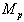is the Planck mass it is noticed that,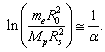(7)
where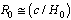and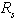is close to 1.5 fm and can be considered as the characteristic nuclear radius or the strong interaction range. Interpretation of this relation seems to be connected with two lengths and two mass units. In this semi empirical relation the most puzzling thing is that, out of the 4 physical LHS parameters, 3 are believed to be fundamental physical constants and they are electron rest mass, Planck mass and the strong interaction range. The only variable is Hubble length. In RHS, the output physical constant is the fine structure ratio. Here interpretation seems to be a sensitive and critical task.

#### 2.3. Application-3

If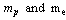are the rest masses of proton and electron respectively, it is noticed that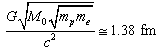(8)
This obtained length is close to the strong interaction range. Whether it is the strong interaction range or something else, is not clear. Here in RHS, the coefficient 2 is missing. From unification point of view this relation can be given a chance either in quantum chromo dynamics or in string theory. From the above two applications, it is possible to say that, the Hubble length plays a key role in atomic and nuclear physics.

#### 2.4. Application-4

Another peculiar relation can be expressed in the following way.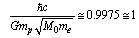(9)
This can be obtained from relations (7) and (8). If this is merely a coincidence, it is very good and the matter ends here. This relation seems to be a mysterious and confusing one. This relation can be analysed in different angles. Its applications seem to be very mysterious. With the above relation, obtained value of the present Hubble’s constant is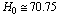Km/sec/Mpc. Now the ‘Bohr radius of hydrogen’ atom can be expressed as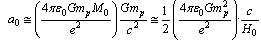(10)
This relation is free from the famous constant h(cross). If nuclear mass increases as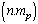where n =1,2,3,.. it is very simple to understand the integral nature of angular momentum. Above relation takes the following form.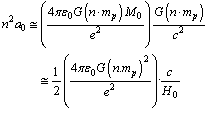(11)
From all these relations it can be interpreted that, in the presently believed atomic and nuclear “physical constants”, there exists one cosmological variable! By observing its cosmological rate of change, the “future” cosmic acceleration can be verified. Thus independent of the cosmic redshift and CMBR observations, with these coincidences it is possible to understand and decide the cosmic geometry. Now in a very simple way, h(cross) and Planck’s constant can be expressed as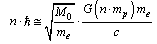(12)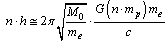(13)
In this way, in a very simplified manner, the integral nature of angular momentum can be understood. This interpretation seems to be quite interesting but at the same time it is very difficult to accept this observation. Considering any two consecutive integers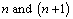, their geometric mean state can be expressed as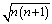and it seems to be the base for the vector atom model. The fine structure ratio can be expressed as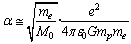(14)
Note that Einstein who understood most of the implications of the emerging interpretations of quantum mechanics, could never accept it as a final theory of physics. He had no doubt that it worked, that it was a successful interim theory of physics, but he was convinced that it would be eventually replaced by a deeper, deterministic theory.

#### 2.5. Application-5

With reference to the Planck mass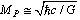(15)
and the elementary charge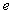, a new mass unit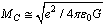(16)
can be constructed. With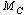and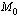it can be assumed that, cosmic thermal energy density, matter energy density and the critical energy density are in geometric series and the geometric ratio is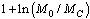Thus,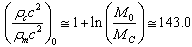(17)
where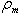is the cosmic matter density.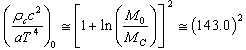(18)
At present, these relations take the following trial-error form: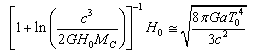(19)
From this relation, if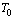is known, by trial–error, present value of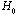can be estimated. With reference to the present CMBR temperature, obtained value of the present Hubble’s constant is close to 71 Km/sec/Mpc. Note that, obtained matter densitycan be compared with the elliptical and spiral galaxy matter density. Based on the average mass-to-light ratio for any galaxy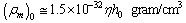(20)
where for any galaxy, M/LGalaxy = ηM/LSun and the number: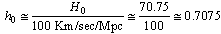.
Note that elliptical galaxies probably comprise about 60% of the galaxies in the universe and spiral galaxies are thought to make up about 20% of the galaxies in the universe. Almost 80% of the galaxies are in the form of elliptical and spiral galaxies. For spiral galaxies, ηh0-1 ≅ 9 ± 1 and for elliptical galaxies, ηh0-1 ≅ 10 ± 2. For our galaxy inner part, ηh0-1 ≅ 6 ± 2. Thus the average ηh0-1 is very close to 8 to 9 and its corresponding matter density is close to (6.0 to 6.67) 10-32 gram/cm3.

### 3. Discussion and Conclusions

Hubble initially interpreted red shifts as a Doppler effect, due to the motion of the galaxies as they receded for our location in the Universe. He called it a ‘Doppler effect’ as though the galaxies were moving ‘through space’; that is how some astronomers initially perceived it. This is different to what has now become accepted but observations alone could not distinguish between the two concepts. Later in his life Hubble deviated from his earlier interpretation and said that the Hubble law was due to a hitherto undiscovered mechanism, but not due to expansion of space - now called ‘cosmological expansion’. This is a very important point to be noted here. With reference to the noticed semi empirical relations - the observed cosmic red shifts can be interpreted in the following way.
1) During the cosmic evolution, the magnitude of Planck’s constant increases and the quantum of energy gradually increases. At present, at all galaxies (either aged or younger), value of Planck’s constant is same. Based on the current concepts of spatial variation of the fine structure ratio, this proposal may be given a chance and may not be ignored.
2)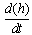is a measure of cosmic rate of expansion. It may be noted that, as the universe is accelerating, value of Planck’s constant increases. Thus if there is no change in the magnitude of Planck’s constant, it can be suggested that, at present there is no cosmic acceleration.
3) During journey light quanta will not lose its energy.
4) Past light quanta emitted from aged galaxy will have less Planck’s constant and show a red shift with reference to the receiving younger galaxy.
Every day quantum mechanics is strongly connected with the constancy of Planck’s constant. String theory, quantum cosmology, quantum chromodynamics (QCD) etc. are strongly based on the constancy of Planck’s constant. With reference to the present concepts of cosmic acceleration and with laboratory experiments one may not decide whether universe is accelerating or decelerating. Many experiments are under progress to detect and confirm the existence of dark matter and dark energy. Along with these experiments if one is willing to think in this new direction, from atomic and nuclear inputs it may be possible to verify the future cosmic acceleration.
With the proposed concepts and with the advancing science and technology, from the ground based laboratory experiments, from time to time the conceptcan be put for experimental tests. There is no need to design a new experiment. Well established experiments are already available by which Planck’s constant can be estimated. Moreover, conducting an experiment in this direction is also very simple. Only thing is that the same experiment has to be repeated for several times or continuously. This is also very simple. Thus in the near future one can expect the real picture.
Alternatively in a theoretical way, the proposed five applications or semi empirical relations can be given a chance and the subject of elementary particle physics and cosmology can be studied in a unified manner. It is true that the proposed relations are speculative and peculiar also. By using the proposed relations and applying them in fundamental physics, in due course their role or existence can be verified. With these relations, Hubble constant can be estimated from atomic and nuclear physical constants. If one is able to derive them with a suitable mathematical model, independent of the cosmic redshift and CMBR observations, the future cosmic acceleration can be verified from atomic and nuclear physical constants. Now the new set of proposed relations are open to the science community. Whether to consider them or discard them depends on the physical interpretations, logics, experiments and observations. In most of the critical cases, ‘time’ only will decide the issue. The mystery can be resolved only with further research, analysis, discussions and encouragement.

### ACKNOWLEDGEMENTS

The first author is indebted to professor Shri K. V. Krishna Murthy, Chairman, Institute of Scientific Research on Vedas (I-SERVE), Hyderabad, India and Shri K. V. R. S. Murthy, former scientist IICT (CSIR) Govt. of India, Director, Research and Development, I-SERVE, for their valuable guidance and great support in developing this subject.

### References

  Hubble E. P, “A relation between distance and radial velocity among extra-galactic nebulae”, PNAS, 1929, ol. 15, 1929, pp.168-173.  J. Huchara. “Estimates of the Hubble Constant”, 2010. Harvard-Smithsonian Center for Astrophysics. http://hubble.plot.dat  W. L. Freedman et al. “Final Results from the Hubble Space Telescope Key Project to Measure the Hubble Constant”. The Astrophysical Journal 553 (1): 47-72.  Seven-Year Wilson Microwave Anisotropy Probe (WMAP) Observations: Sky Maps, Systematic Errors, and Basic Results. Nasa.Gov. Retrieved 2010-12-02.  Geiger H and Marsden E. “On a diffuse reaction of the particles”. Proc. Roy. Soc., Ser. A 82: 495-500, 1909.  P. A. M. Dirac. “The cosmological constants”. Nature, 139, 323, 1937.  P. A. M. Dirac. “A new basis for cosmology”. Proc. Roy. Soc. A 165, 199, 1938  J.K. Webb et al. “Indications of a spatial variation of the fine structure constant”. Physical Review letters, 107 (19) 2011.  David Gross, “Einstein and the search for Unification”. Current science, Vol. 89, No. 12, 25 Dec 2005.  J.V. Narlikar, Introduction to cosmology, Cambridge Univ. Press, 2002, 393 – 400.  Hubble, E.P, “The 200-inch telescope and some problems it may solve”. PASP, 59, pp153-167, 1947.  Hawking S.W. A brief history of time. Bantam Dell publishing group.1998.